Constructing tangent when circle's center is not given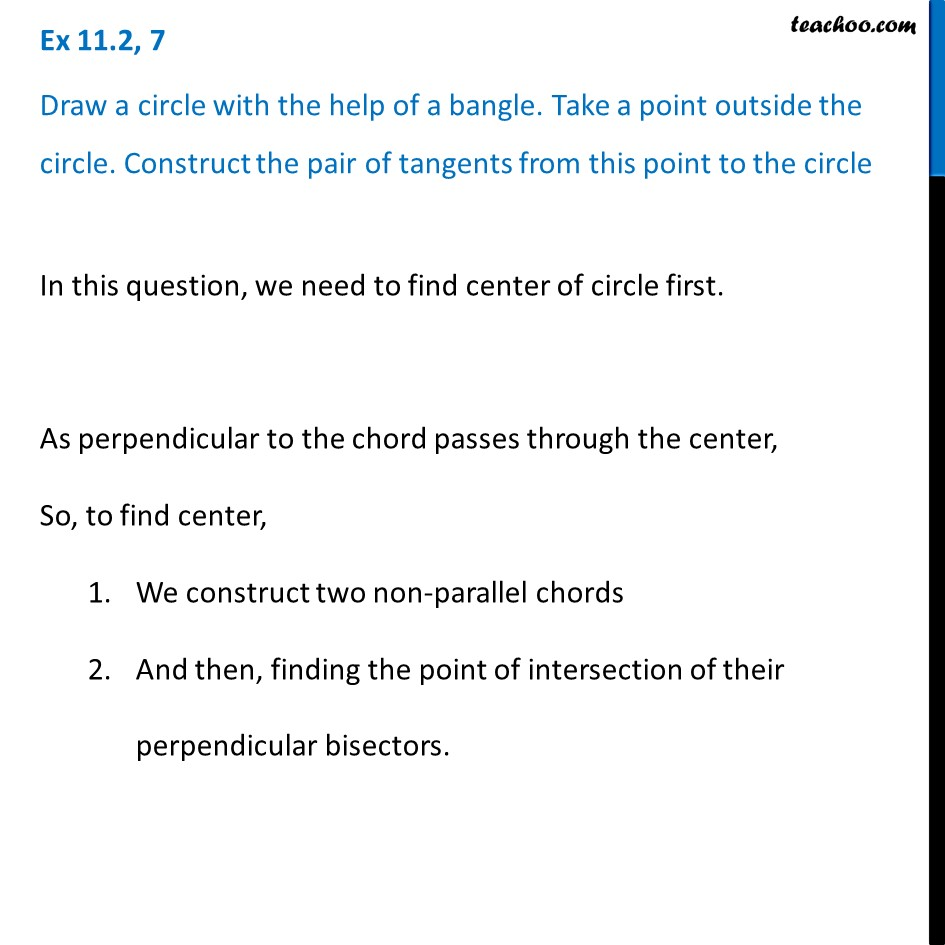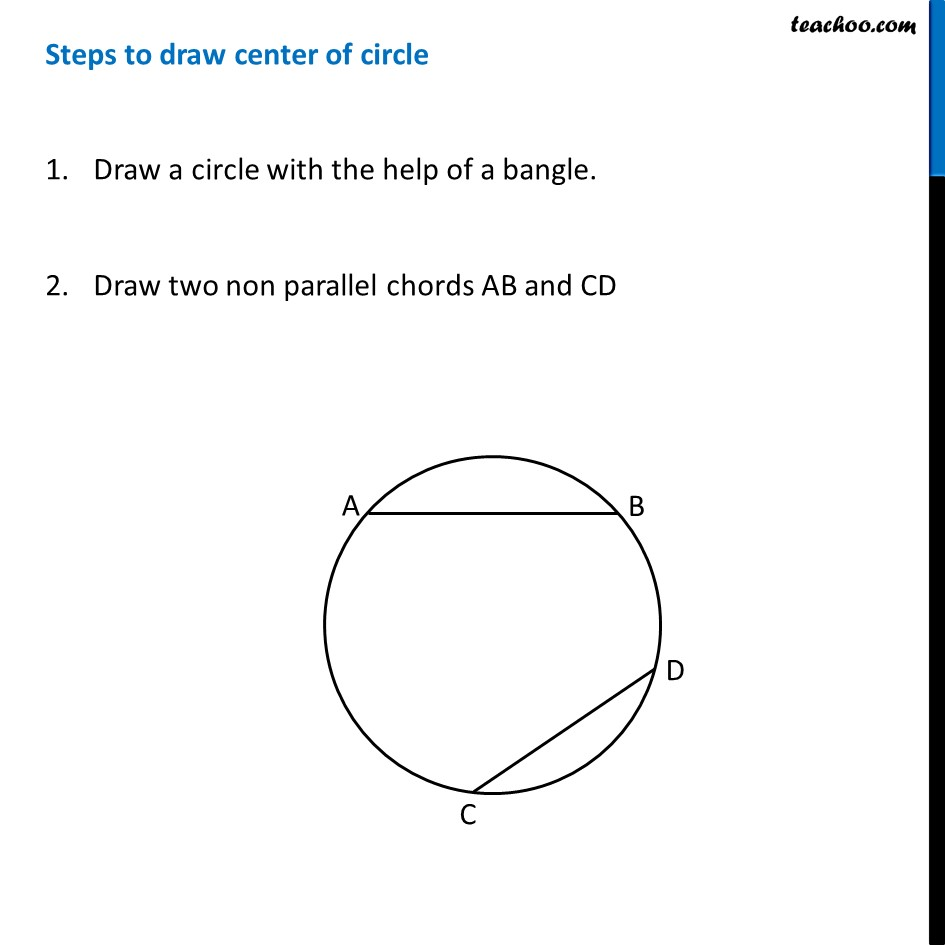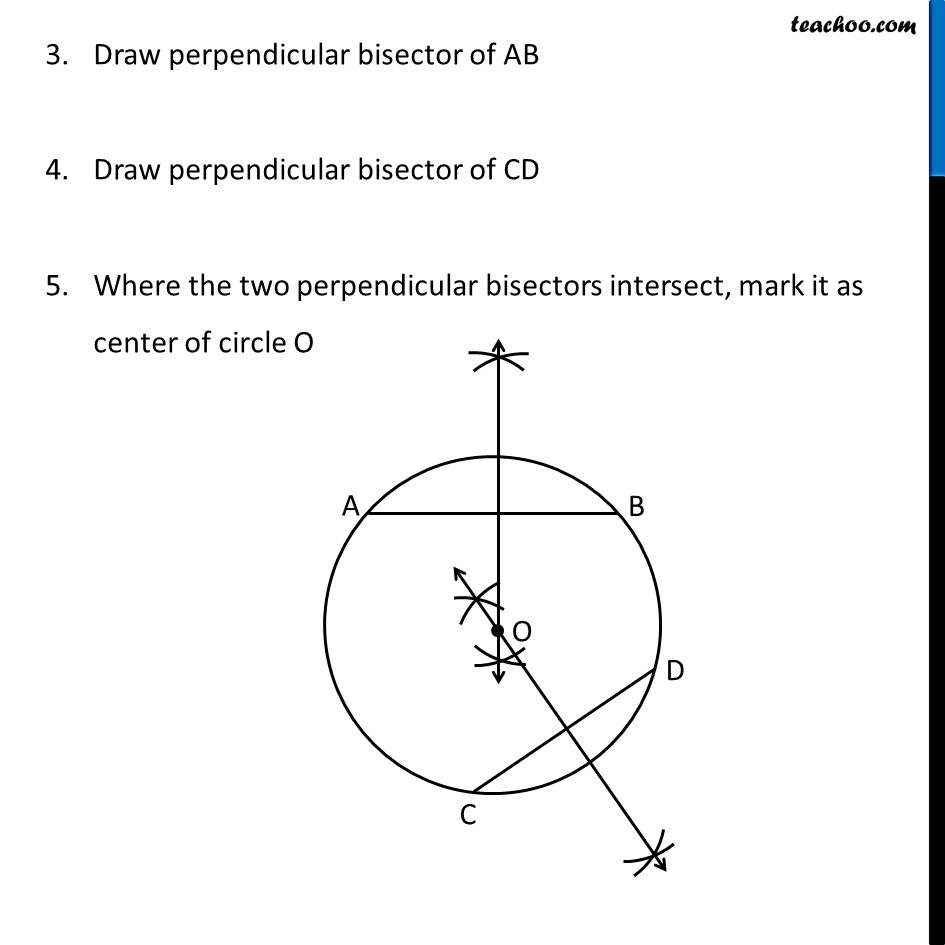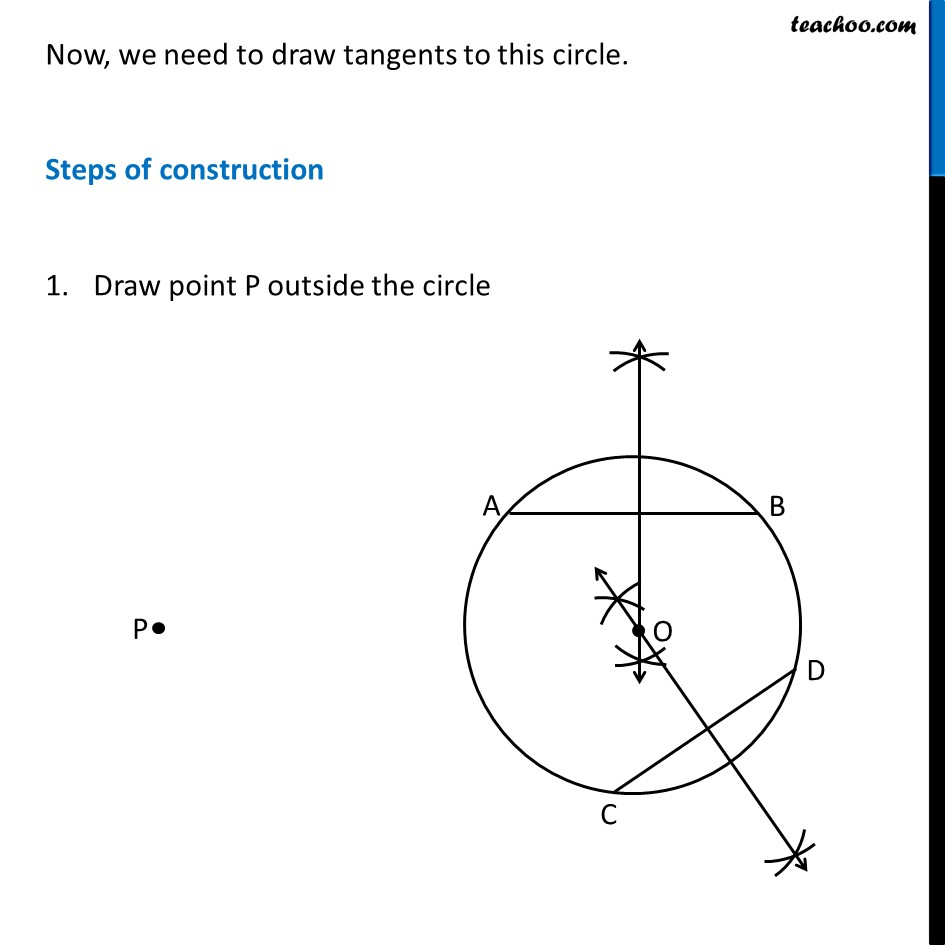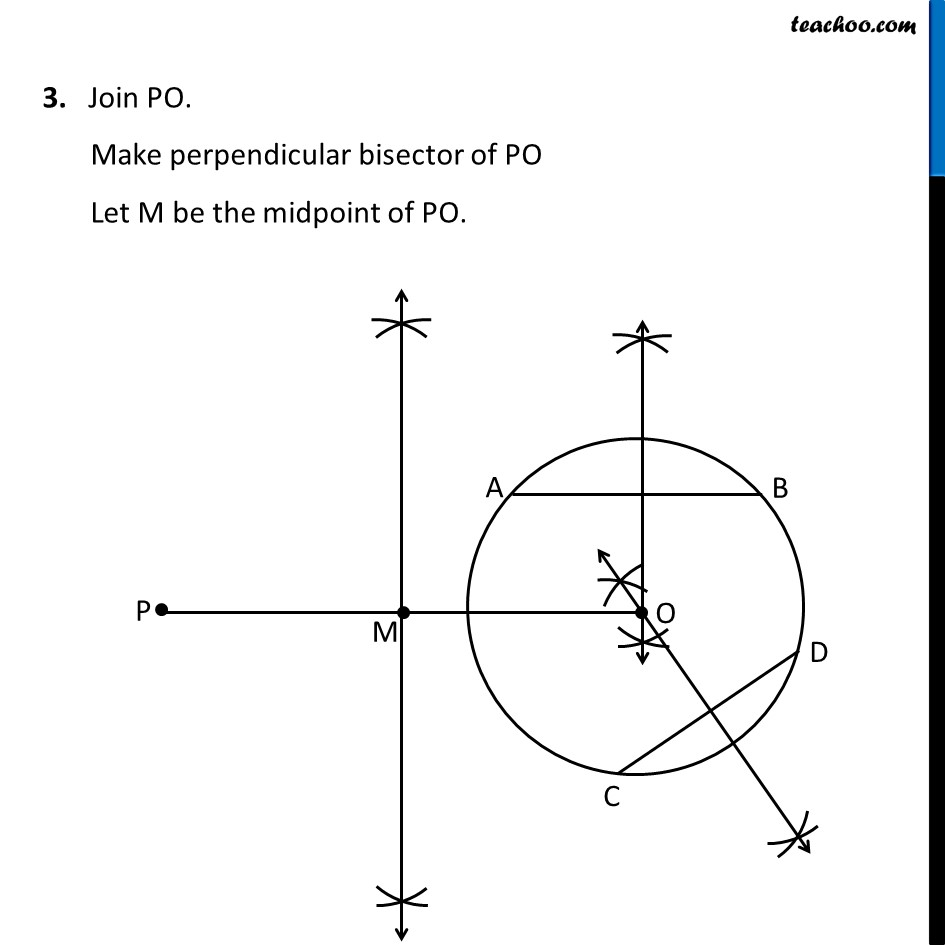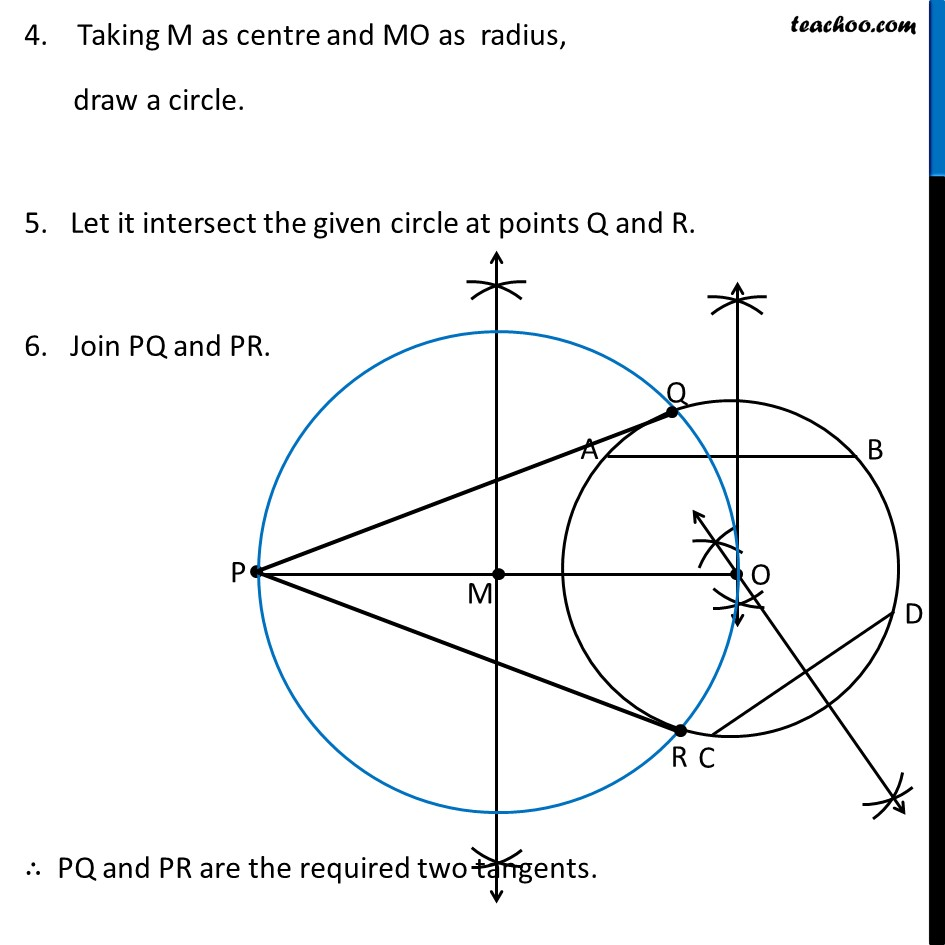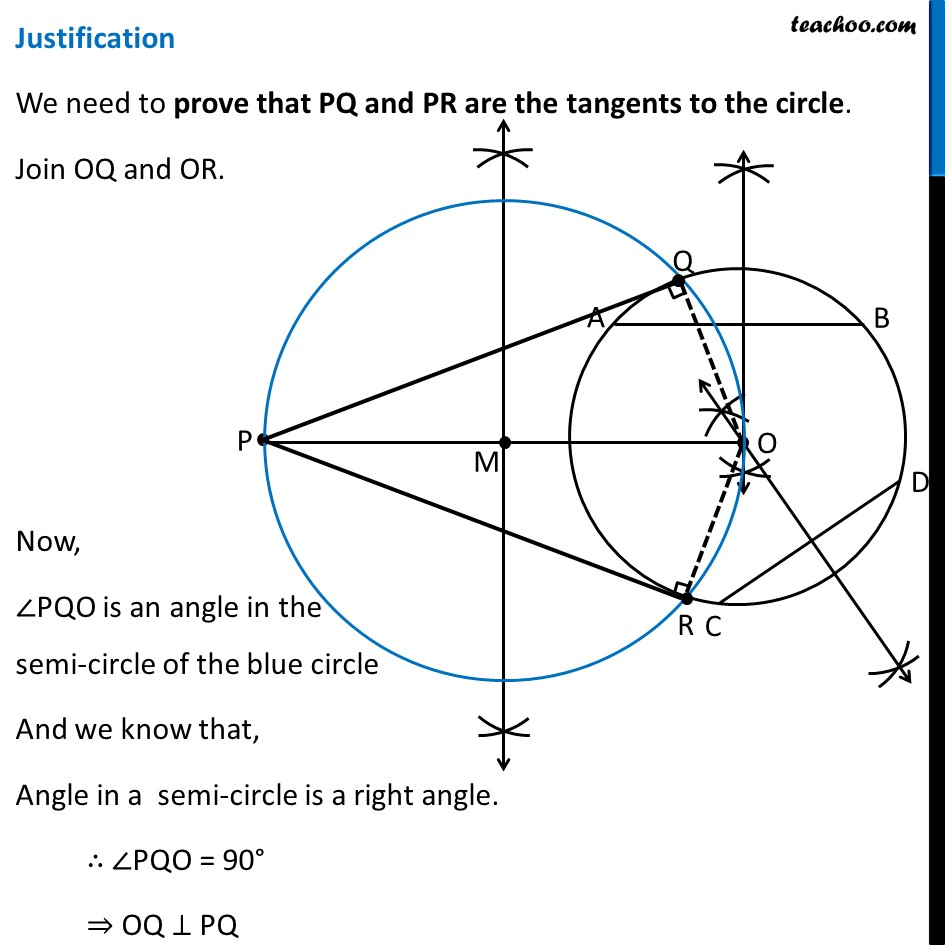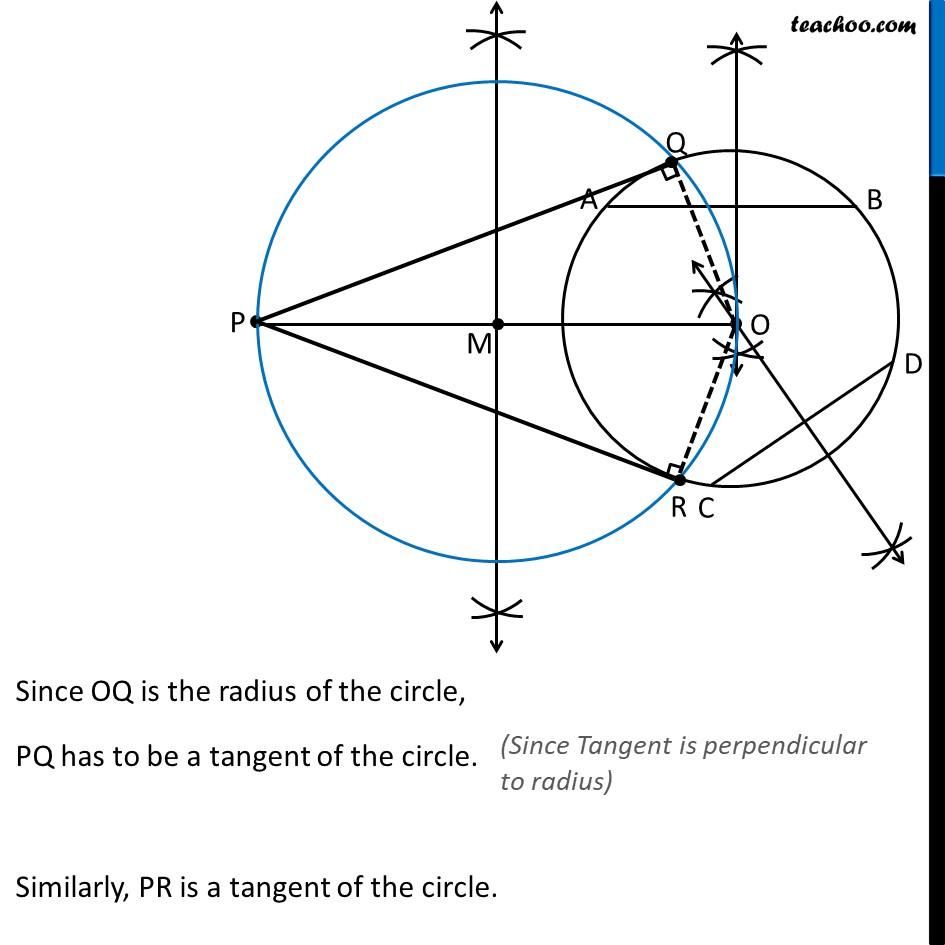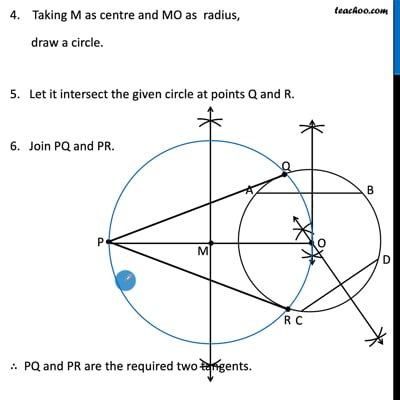This video is only available for Teachoo black users

Introducing your new favourite teacher - Teachoo Black, at only ₹83 per month

### Transcript

Ex 11.2, 7 Draw a circle with the help of a bangle. Take a point outside the circle. Construct the pair of tangents from this point to the circle In this question, we need to find center of circle first. As perpendicular to the chord passes through the center, So, to find center, We construct two non-parallel chords And then, finding the point of intersection of their perpendicular bisectors. Steps to draw center of circle Draw a circle with the help of a bangle. Draw two non parallel chords AB and CD Draw perpendicular bisector of AB Draw perpendicular bisector of CD Where the two perpendicular bisectors intersect, mark it as center of circle O Now, we need to draw tangents to this circle. Steps of construction Draw point P outside the circle 3. Join PO. Make perpendicular bisector of PO Let M be the midpoint of PO. 4. . Taking M as centre and MO as radius, draw a circle. 5. Let it intersect the given circle at points Q and R. 6. Join PQ and PR. ∴ PQ and PR are the required two tangents. Justification We need to prove that PQ and PR are the tangents to the circle. Join OQ and OR. Now, ∠PQO is an angle in the semi-circle of the blue circle And we know that, Angle in a semi-circle is a right angle. ∴ ∠PQO = 90° ⇒ OQ ⊥ PQ Since OQ is the radius of the circle, PQ has to be a tangent of the circle. Similarly, PR is a tangent of the circle. (Since Tangent is perpendicular to radius)